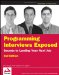# Chapter 11: Other Programming Topics

A couple of interview topics are less common than those we’ve looked at so far. These topics do appear frequently enough in interviews, though, to merit discussion. The first topic is graphics, and the second is bit manipulation. The latter topic in particular often occurs early in an interview as a warm-up to the more-challenging problems.

## Graphics

A computer screen consists of pixels arranged in a Cartesian coordinate system. This is commonly called a raster pixel display. Computer graphics algorithms change the colors of sets of pixels. Often, the algorithm for generating a raster pixel image is based on a geometric equation. Because a computer screen has a finite number of pixels, translating from a geometric equation to a pixel display can be quite complex. Geometric equations usually have real-number (floating-point) solutions, but pixels are found only at fixed, regularly spaced locations. Therefore, every point that is calculated must be adjusted to pixel coordinates. This requires some kind of rounding, but rounding to the nearest pixel coordinate is not always the correct approach. It is often necessary to round numbers in unusual ways or add error-correcting terms. When rounding is done carelessly, it often leads to gaps in what should be continuous lines. Take care to check your graphics algorithms for distortion or gaps due to poor rounding or error correction.

Consider something as simple as drawing a line segment, for example. Suppose you were trying to implement a function that takes two endpoints and draws a line between them. After doing a little algebra, you could easily get an equation in the form of y = mx + b. Then, you could calculate y for a range of x values and draw the points making up the line. This function seems trivial.

The devil, though, is in the details of this problem. First, you must account for vertical lines. In this case, m is infinity, so the simple procedure can’t draw the line. Similarly, imagine that the line is not vertical, but close to vertical. For example, suppose that the horizontal distance spanned by the line were 2 pixels, but the vertical distance were 20 pixels. In this case, only two pixels would be drawn - not much of a line. To correct for this problem, you would have to rework your equation to x = (y – b) / m. Now, if the line is closer to vertical, then you vary y and use this equation; if it is closer to horizontal, then you use the original procedure.

Even this won’t solve all your problems. Suppose you need to draw a line with a slope of 1 - for example, y = x. In this case, using either procedure, you would draw the pixels (0, 0), (1, 1), (2, 2). This is mathematically correct, but the line looks too thin on the screen because the pixels are much more spread out than in other lines. A diagonal line of length 100 has fewer pixels in it than a horizontal line of length 80. An ideal line-drawing algorithm would have some mechanism to guarantee that all lines have nearly equal pixel density.

Another problem involves rounding. If you calculate a point at (.99, .99) and use a type cast to convert this to integers, then the floating-point values will be truncated and the pixel will be drawn at (0, 0). You need to explicitly round the values so that the point is drawn at (1, 1).

If graphics problems seem like never-ending series of special cases, then you understand the issues involved. Note that even if you were to work out all the problems with the line-drawing algorithm described, it still wouldn’t be very good. Although this algorithm effectively illustrates the problems encountered in graphics programming, its reliance on floating-point calculations makes it very slow. High-performance algorithms that use only integer math are far more complicated than what is discussed here.

 Tip Computer graphics involves drawing with pixels. Always check for rounding errors, gaps, and special cases.Programming Interviews Exposed: Secrets to Landing Your Next Job, 2nd Edition (Programmer to Programmer)
ISBN: 047012167X
EAN: 2147483647
Year: 2007
Pages: 94

Similar book on Amazon

flylib.com © 2008-2017.
If you may any questions please contact us: flylib@qtcs.net